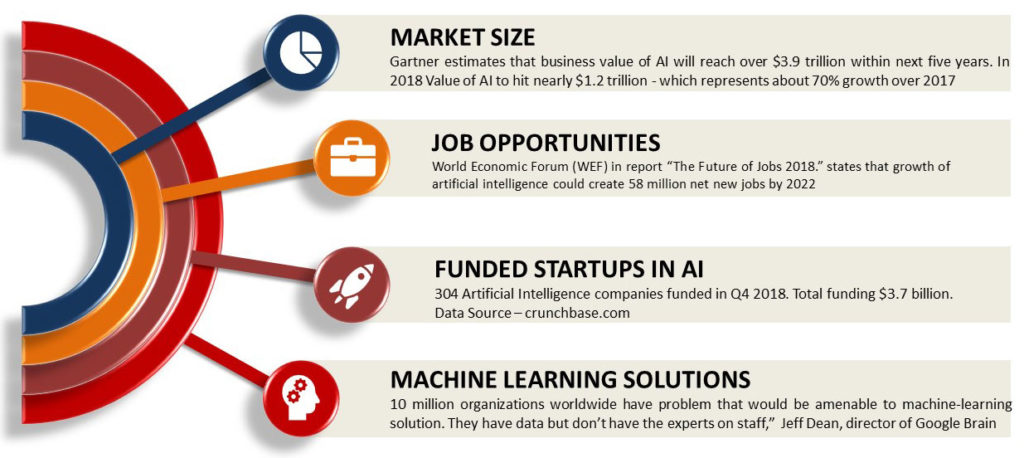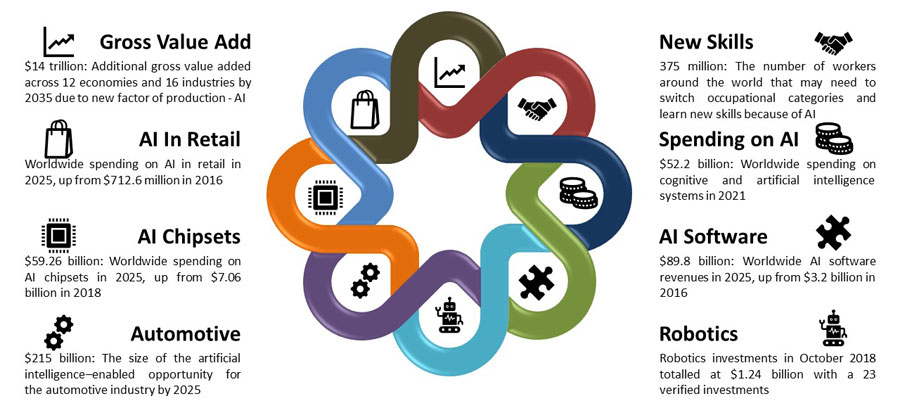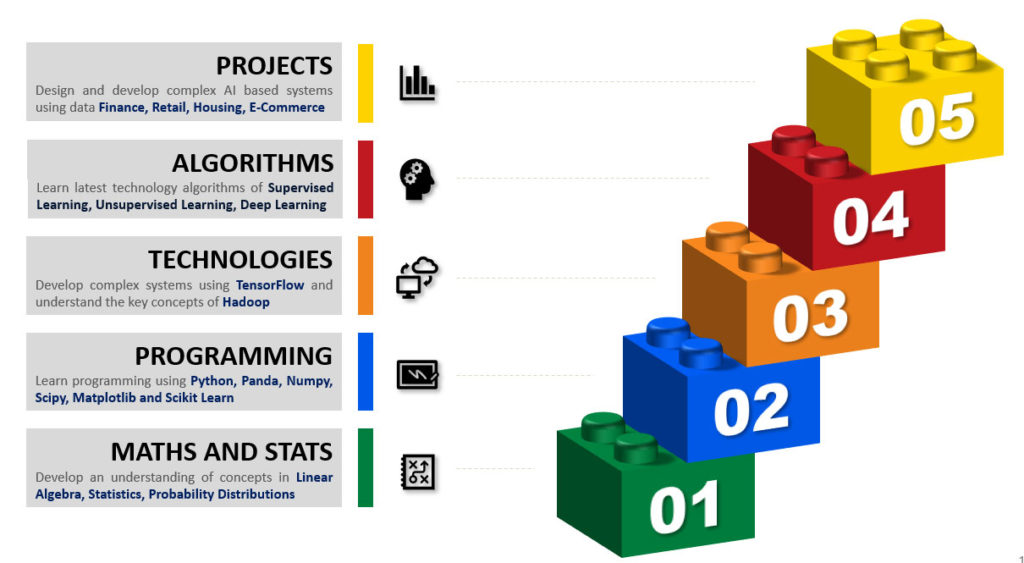# Artificial Intelligence Machine Learning Deep Learning With Python

## From Leading AI Training Providers

Upskill with Big Data Learning Program
Contact for more details

## Experienced and qualified facultyClassroom sessions conducted by IIT, IIM alumni with 40 years of industry experience

## Focus on concepts with practicalsEmphasis on concepts of Data Analysis with Python, statistics, linear algebra, machine learning, and deep learning

## Instructor led classroom sessions160+ hour of instructor-led classroom sessions. Additional 250+ hours for projects and assignments

## Virtual classroom sessionsOption of attending the session from home using video conference. Join live classroom and participate in discussion

## Artificial Intelligence MarketWe usually take decisions based on the information. On several occasion, we take the decision based on data and on another occasion, we take decision-based on experience and intuition. In either case, we predict future based on information and experience and make decisions based on predictions. Several fields have been developed to take our information, analyze the data and give predictions.  Artificial intelligence, machine learning, data mining, and pattern recognition are some most popular fields. Each of the fields uses different tools and techniques to make an accurate prediction.

The training program in artificial intelligence and machine learning has been designed to learn some of the most advanced methods and learn practical techniques to implement them. The course provides a comprehensive introduction to machine learning and statistical pattern recognition. The course will use several case studies so that you will learn how to apply the statistical methods and learning algorithms in solving real-life problems.

The course will cover linear regression, classification, shrinkage approaches, tree-based models, clustering, support vector machines, resampling methods, neural network and more. From a technology perspective, you will learn python, numpy, matplotlib, panda, scikit-learn, tensorflow, and keras

The artificial intelligence and machine learning program are useful for freshers as well as corporate professionals, who either want to switch career to machine learning or want to build deep expertise in the subject.

In this class, you will learn about a set of tools for understanding and modeling complex data sets. The course is a perfect blend of foundations, theory and hands-on experience.

You will understand the most effective machine learning methods, applications of the methods, and techniques to select the best method for the given datasets. You will practice implementing them and getting them to work for yourself.

The key objective of the artificial intelligence and machine learning program is to bring together many of the tools and techniques together and learn in a statistical and programming framework

## What you will learn?

Key concepts of machine learning, statistical tools, deep learning, neural networks. You will get an opportunity to work on 7 projects.

## Learning Centered PedagogySamatrix pedagogy is learning centered. The curriculum has been designed to develop analytical skills based on mathematics and statistics oriented approach. It will help the learner build the career beyond data analytics and technical jobs.

Our learning model starts with the fundamentals of linear algebra, statistics, probability distributions, python programming. Build on those through deep understanding of machine learning algorithms, learner explores machine learning through some fun problems.

The artificial intelligence and machine learning program also focuses on building the capability to interpret model results, improving and tuning the models, driving to business value using machine learning methods

## Course Curriculum

A cutting-edge curriculum that aligns to industry requirements, globally-recognized standards and best practices

• Systems of Linear Equations
• Row Reduction and Echelon Forms
• Existence and Uniqueness
• Vectors and Matrix Equations
• Vector Matrix Products
• Linear Independence
• Matrix Multiplication
• Transpose of Matrix
• Inverse of Matrix
• Vector Space, Null Space, Row Space
• Eigenvalues and Eigenvectors
• Orthogonality
• Graphically Displaying Single Variable
• Measures of Location
• Mean
• Median
• Range
• Variance
• Standard Deviation
• Displaying relationship – Bivariate Data
• Scatterplot
• Scatterplot Matrix
• Measures of association of two or more variables
• Covariance and Correlation
• Probability
• Joint Probability and independent events
• Conditional probability
• Bayes’ Theorem
• Prior, Likelihood and Posterior
• Discrete Random Variable
• Probability Distribution of Discrete Random Variable
• Binomial Distribution
• Continuous Random Variables
• Probability Distribution Function
• Uniform Distribution
• Normal Distribution
• Beta Distribution
• Point Estimation
• Interval Estimation
• Expectation Theory
• Hypothesis Testing
• Testing a one-sided Hypothesis
• Testing a two-sided Hypothesis
•
• Introduction to Python
• Jupyter
• Numpy
• Matplotlib
• Seaborn
• Panda
• Scikit Learn
• What is Machine Learning
• Machine Learning vs Computer Program
• Define Machine Learning
• Application of Machine Learning
• Relation between variables
• Supervised Learning
• Unsupervised Learning
• Semi-Supervised Learning
• Reinforcement Learning
• Prediction
• Dependent Variable vs Independent Variables
• Reducible Error and Irreducible Error
• Expected Value and Variance
• Inference
• Which Predictors are associated with Response?
• Relationship between response and predictors
• Learning Methods
• Parametric Methods
• Non Parametric Methods
• Model Flexibility vs Interpretability
• Model Accuracy and Selection
• Quality of Fit
• Bias – Variance Trade Off
• Bayes Classifier
• K-Nearest Neighbors
• Basic Concepts
• Construction of Regression Model
• Selection of Predictor Variables
• Functional Form of Regression Relations
• Scope of Model
• Uses of Regression Analysis
• Description
• Control
• Prediction
• Regression and Causality
• Formal Statement of Model
• Important Features of Model
• Meaning of Regression Parameters
• Steps in Regression Analysis
• Estimation of Regression Function
• Least Square Estimator
• Estimating the Coefficients
• Estimation of Variance Terms
• Accuracy of Coefficients
• Accuracy of Model
• Residual Standard Error
• R Square Statistics
• Multiple Linear Regression
• Estimating Regression Coefficients
• Analysis
• Relationship between Response and Predictor
• Important Variables
• Model Fit
• Predictions
• Qualitative Regression Models
• Synergy / Interaction Effect
• Polynomial Regression
• Problems with Regression Models
• Non-linearity of the data
• Correlation of error terms
• Non constant variance of error terms
• Outliers
• Leverage Points
• Collinearity
• Basic Concept with Example
• Why not Linear Regression
• Logistic Regression
• Logistic Model
• Estimating Regression Coefficients
• Multiple Logistic Regressions
• Linear Discriminant Analysis
• Nearest Neighbour Methods
• Cross Validation
• Bootstrap
• Choosing Optimal Model
• F Test
• Likelihood Ratio Test (LRT)
• Akaike Information Criterion (AIC)
• Bayes Information Criterion (BIC)
• Subset Selection
• Best Subset Selection
• Forward Stepwise Selection
• Backward Stepwise Selection
• Ridge Regression
• Ridge Regression vs Least Square
• Lasso Regression
• Variable Selection Property of Lasso
• Singular Value Decomposition (SVD)
• Principal Components
• Principal Component Analysis (PCA)
• Geometric Interpretation
• Polynomial Regression
• Step Functions
• Regression Splines
• Smoothing Splines
• Local Regression
• Construct the Tree
• Regression Tree
• Classification Tree
• Impurity Functions
• Entropy
• Gini Index
• Misclassification Rate
• Tree Pruning
• Bagging
• Random Forests
• Boosting
• Maximal Margin Classifier
• Support Vector Classifier
• Support Vector Machine
• Introduction to Neural Network
• Perceptrons
• NAND Gate
• Sigmoid Neuron
• Multilayer Neural Network
• Architecture of Multilayer Network
• Backward Propagation Algorithm
• Cross Entropy Cost Function
• Overfitting and Regularization
• Weight Initialization
• Meta-Heuristic Optimization
• Simulated Annealing
• Particle Swarm Optimization
• Genetic Algorithms
• Ant Colony Optimization
• Differential Evolution
• Genetic Programming
• Introduction to TensorFlow
• TensorFlow Basics
• Computation Graphs
• Graphs, Sessions and Fetches
• Flowing Tensors
• Variables, Placeholders, and Simple Optimization
• Introduction to Keras
• Introduction to Convolutional Neural Networks
• Introduction to Recurrent Neural Networks (RNN)
• Auto-Encoders
• The Challenge of Unsupervised Learning
• Principal Component Analysis
• Clustering Methods
• K-Mean Clustering
• Hierarchical Clustering
• Practical Issues in Clustering

## Key Learning Areas

The learning areas include hands-on expertise in machine learning, deep learning, and models such as Convolutional Neural Networks, Recurrent Neural Networks. The program focuses on building expertise in  data analysis, machine learning and neural network concepts. Even if you do not have any expertise in machine learning and data analysis, you will learn faster from our classes.

Data Analysis
Using Python, pandas, statistics, linear algebra
Machine Learning
Regression, Classification, unsupervised learning
Deep Learning
Neural Networks, Deep learning models with TensorFlow, Keras

## Selection Process

1. Fill Application Form: Apply online using the application form
2. Application Review: Admission committee review the applications submitted
3. Personal Interview: The Admission committee can invite the applicant for a personal interview.

## Placement Assistance Program

Samatrix has a dedicated placement assistance team. Under our placement assistance program, we help the learners, who enroll for the program, introduce to our 100+ hiring partners.

On successful completion of the training requirements, the learner becomes eligible for the training assistance program. Our training assistance team work closely with the learners to understand their career goals and provide mentorship.

We prepare the learners for application process and interviews by conducting resume review workshops, mock HR and technical interviews with industry experts.

## Join the training program

Learn the latest technology and be part of a revolution.

Training Program Fee Rs 65000 + GST

Registration Fee Rs 15000

The training program has been designed for both fresher and professionals with industry experience. If the learner has the willingness to learn and dedication to spend time, he/she will certainly benefit from the program

This is an instructor-led classroom face to face training program. Face to face classroom training helps learners focus on the training program and provides an opportunity to interact with the trainer and other participants.

After successful completion of training program and projects, Samatrix.io will issue a certificate of completion of training

Several industry leaders are hiring partners of Samatrix.io. Our team would help all the learners in job placement. Majority of our trainees have been successfully placed.

The curriculum of Artificial Intelligence Machine Learning training program is very rigorous. This is 135+ hours of instructor led program. The program will start at a designated time on weekends over 4 months. During the course, you will have access to one of the best study material that includes:

1. Powerpoint presentations
2. Recording of the sessions
3. Case study with dataset
4. Any other relevant study material on the topic

Each learner will have his personal userid through which he can access the study material through the learning management system. Each learner will be provided the access to the LMS for 1 year including course duration